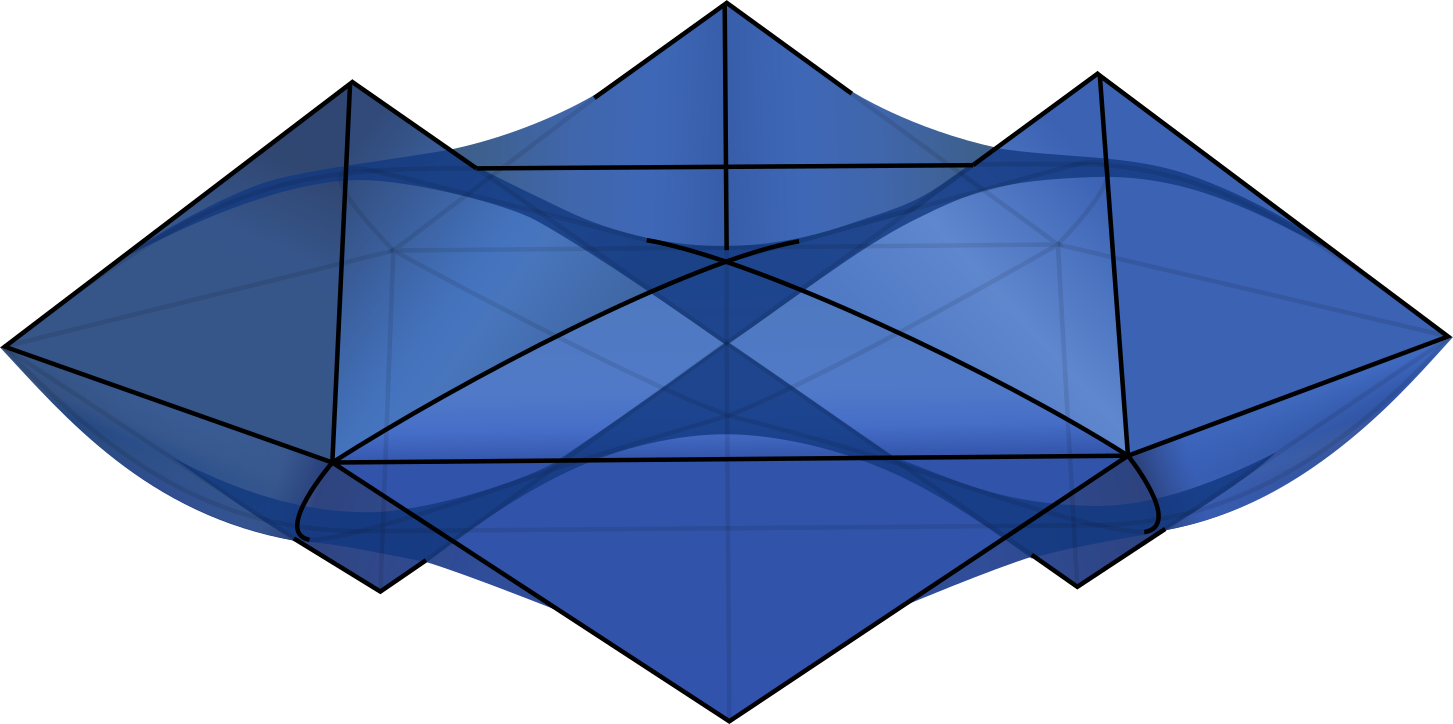# Intersection Bodies of Polytopes¶

Katalin Berlow, Marie-Charlotte Brandenburg, Chiara Meroni, and Isabelle Shankar: Intersection bodies of polytopes
In: Beiträge zur Algebra und Geometrie, 63 (2022) 2, p. 419-439Abstract: We investigate the intersection body of a convex polytope using tools from combinatorics and real algebraic geometry. In particular, we show that the intersection body of a polytope is always a semialgebraic set and provide an algorithm for its computation. Moreover, we compute the irreducible components of the algebraic boundary and provide an upper bound for the degree of these components.

We now present an implementation of Algorithm 1 in the paper, written in $$\verb|SageMath|$$ 9.2.

## Implementation of Algorithm 1¶

We offer predefined functions for $$\verb|SageMath|$$ and $$\verb|Oscar|$$.

### SageMath¶

For $$\verb|SageMath|$$, download the file intersection_body_functions.py and place them in a folder together with a new SageMath Notebook or Jupyter Notebook. In the notebook, first define your favourite polytope P, such as

P = polytopes.cube()


Then run the following technical lines:

from intersection_body_functions import *
variables = [var("x"+str(i)) for i in range(1,P.dimension()+1)]


Finally, we can use the predefined functions radial_function_IP and algebraic_boundary as follows

rho = radial_function_IP(P,variables)


While the function is computing the radial function, it produces some output and tells you when it is done:

> computing hyperplane arrangement...
> completing it to a fan...
> checking if the origin is inside the polytope...
> 0 of 14
> 10 of 14
> done


To compute the algebraic boundary from the radial function, simply type

alg_boundary(rho)


The output of radial_function is a list of pairs, each pair consists of an expression $$\rho(x)=\frac{p(x)}{\|x\|^2 q(x)}$$ and the cone $$C$$ from the hyperplane arrangement on which the expression is valid. These objects are described in more detail in Section 2 of the paper.

The output of alg_boundary is again a list of pairs, where now each pair consists of a polynomial that describes the algebraic boundary of the intersection body and the cone $$C$$.

### OSCAR¶

The jupyter notebook Intersection_bodies_OSCAR.ipynb contains the predefined functions similar to the functions described above for $$\verb|SageMath|$$. It also contains a step by step explanation that is analogous to the one for $$\verb|SageMath|$$ mentioned below. Note that this current implementation for $$\verb|Oscar|$$ only computes itersection bodies of 3-dimensional polytopes.

## Understanding the algorithm step by step¶

On this page, we present how the functions radial_function_IP and alg_boundary operate step by step, on the example of the 3-dimensional cube. For simplicity, we omit the triangulations that are needed for polytopes of higher dimension, as well as technicalities that arise when the origin does not lie in the interior of the polytope. These steps are explained in more detail in Sections 3 and 4 of the paper. To download the Jupyter notebook that is displayed on the page, please click here.

## What is the role of the origin?¶

Are you wondering about the role of the origin in the construction of intersection bodies? In this gallery we explore the different shapes of intersection bodies of the 3-cube, depending on the position of the origin relative to the cube.

Credits
Project page created: 01/10/2021
Project contributors: Katalin Berlow, Marie-Charlotte Brandenburg, Chiara Meroni, and Isabelle Shankar
Software used: SageMath (Version 9.2), Julia (Version 1.7.1), OSCAR (Version 0.8.2-DEV)
System setup used: MacBook Pro with macOS Monterey 12.0.1, Processor 2,8 GHz Quad-Core Intel Core i7, Memory 16 GB 2133 MHz LPDDR3, Graphics Intel HD Graphics 630 1536 MB.